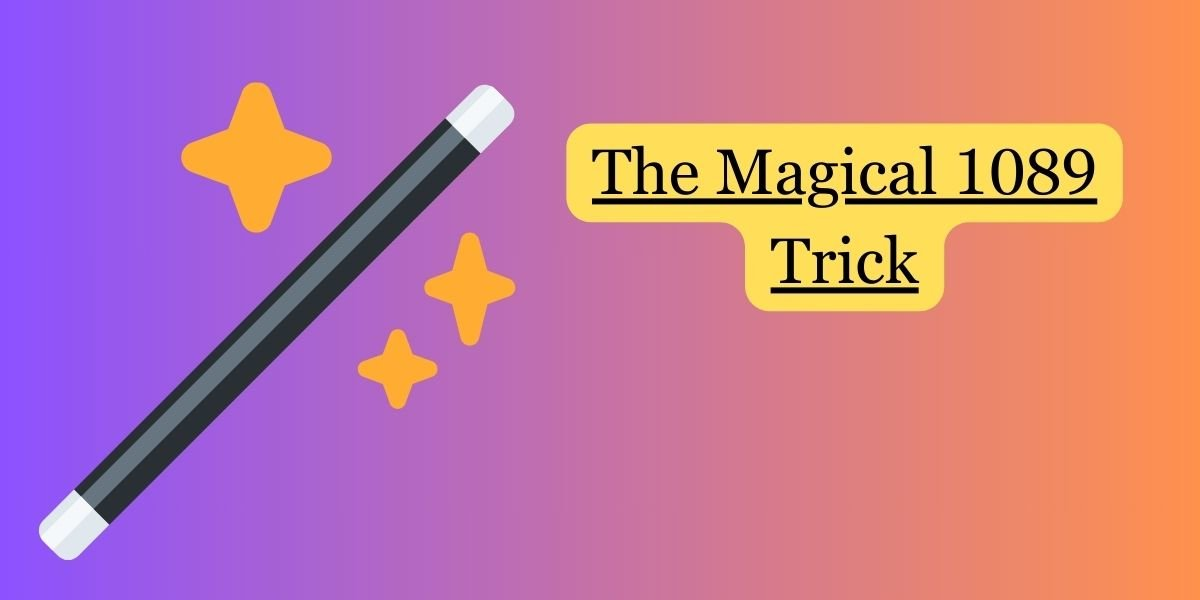Steps:

1. Ask someone to choose any three-digit number. For example, let’s say they choose 724.
2. Arrange the digits to form two new numbers, one in ascending order and the other in descending order.
• Ascending order: 247
• Descending order: 742
3. Subtract the smaller number from the larger one.
• 742 – 247 = 495
4. Reverse the result and add it to the original result.
• 495 + 594 = 1089

The Magic: No matter what three-digit number the person initially chooses and follows the steps, the result will always be 1089!

Explanation: The trick works because of the properties of numbers and basic arithmetic. The key is in the subtraction step. When you subtract a smaller number from a larger one, you always get a result with the digits rearranged in a specific way. Reversing and adding this result consistently leads to the magic number 1089.

Performing this trick can add an element of wonder to basic math concepts and make it an engaging experience for kids and adults alike.